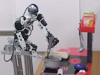### Abstract

Manipulation tasks are characterized by continuous motion trajectories containing a set of key phases. In this paper, we propose a probabilistic method to autonomously segment the motion trajectories for estimating the key phases embedded in such a task. The autonomous segmentation process relies on principal component analysis to adaptively project into one of the low-dimensional subspaces, in which a Gaussian mixture model is learned based on Bayesian information criterion and expectation-maximization algorithms. The basis skills are estimated by a set of Gaussians approximating quasi-linear key phases, and those times spent calculated from the segmentation points between two consecutive Gaussians representing the local changes of dynamics and directions of the trajectories. The basis skills are then used to build novel motion trajectories with possible motion alternatives and optional parts. After sequentially reorganizing the basis skills, a Gaussian mixture regression process is used to retrieve smooth motion trajectories. Two experiments are presented to demonstrate the capability of the autonomous segmentation approach.

### Bibtex reference

@inproceedings{Lee12Hum,
author="Lee, S. H. and Suh, I. H. and Calinon, S. and Johansson, R.",
title="Learning Basis Skills by Autonomous Segmentation of Humanoid Motion Trajectories",
booktitle="Proc. {IEEE} Intl Conf. on Humanoid Robots ({H}umanoids)",
year="2012",
}